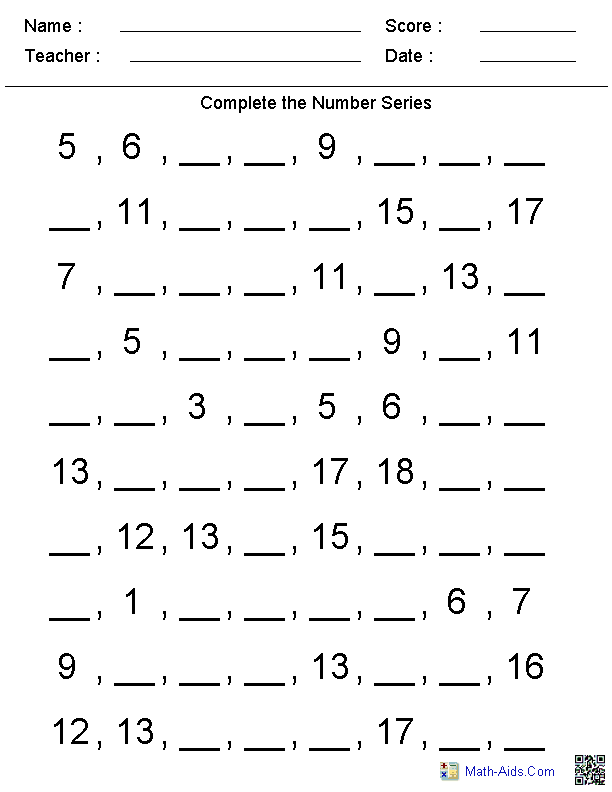## lbartman.com - the pro math teacher

• Subtraction
• Multiplication
• Division
• Decimal
• Time
• Line Number
• Fractions
• Math Word Problem
• Kindergarten
• a + b + c

a - b - c

a x b x c

a : b : c

# Kindergarten Math Worksheet

Public on 04 Oct, 2016 by Cyun Lee

###kindergarten worksheets dynamically created kindergarten worksheets

Name : __________________

Seat Num. : __________________

Date : __________________

### HOW MANY STARS EACH LINE ?

......
......
......
......
......
show printable version !!!hide the show

## RELATED POST

Not Available

## POPULAR

free printable multiplication color by number worksheets

maths word problems worksheets

multiplying and dividing algebraic fractions worksheet

first grade math common core worksheets

addition and subtraction word problem worksheets

october math worksheets

multiplication worksheet